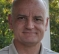## Removing a known noise component from a signalStarted by 5 years ago3 replieslatest reply 5 years ago248 views

I have been working on a problem that in theory should be easy to solve but in practice has turned into the diametric opposite.The context of the problem is that of filtering load cell force time history data from vehicle to fixed, rigid, massive barrier collision testing conducted under the auspices of the National Highway Traffic Safety Administration (front of test vehicle to barrier in a collinear impact configuration).  The barrier, itself, consists of K individual load cells (K generally being 8, 36 or 128).  The event, itself, consists of the collision between the test vehicle and the barrier.For any given test, the start of the closure phase of the collision (i.e. the first moment of contact between the test vehicle and the barrier) is established independently by high-speed video or tapeswitch.The data generated from any given test typically includes pre-event data of roughly 20 milliseconds in length.In theory, the pre-event data should be zero-valued in regards to force amplitude due to zeroing of the load cells.In practice, however, this is rarely the case.In certain cases, the source of the non-zero valued pre-event data is readily deterministic (e.g. contact between the towline and the barrier) but not in all cases.

The following serves as an example (NHTSA Test number v03196):

The sample rate for all load cells was 12.5 kHz.The load cells for the barrier were arranged in four rows of nine load cells.The pre-event data (force v. time) for the leftmost three load cells of the bottom two rows is shown in Figure 1 (each red dot denotes an individual sample).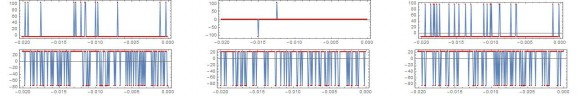Figure 1.  Force-time history pre-event plots for load cells A1-3 (top row as shown) and B1-3 (bottom row as shown).

The power spectrum for the pre-event data for the first load cell is shown in Figure 2 and for the entire signal from the same load cell (i.e. pre-event and event data) is shown in Figure 3.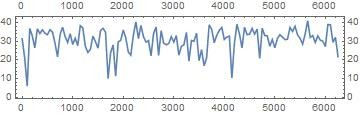Figure 2.  Power spectrum for the pre-event portion of the data from load cell A1.Figure 3.  Power spectrum for the entirety (pre-event and event data) of the data from load cell A1.

I have attempted to see if determining the distribution of the pre-event data (Figure 4) and the entire time series (pre-event and event data) (Figure 5) would provide some guidance but it has not.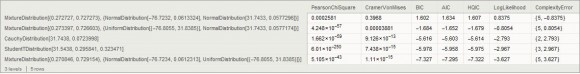Figure 4.  Distribution fits for the pre-event data from load cell A1.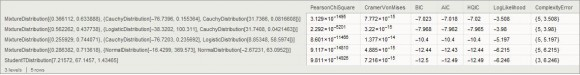Figure 5.  Distribution fits for the entirety (pre-event and event data) of the data from load cell A1.

The current standard for filtering this kind of data is set in the SAE J211 standard and consists of employing a Butterworth four pole phaseless digital filter (Channel frequency class 60 filter).  The use of the pre-event data for filter start-up or padding by means of adding the transpose (about the time and magnitude axes) of at least 10 milliseconds of event data still results in non-mitigation of the general problem of a non-zero valued force at the start of the event.This particular issue is problematic in that the dynamic center of mass acceleration of the test vehicle is calculated from the sum of the barrier load cell data, normalized by the mass of the test vehicle.The acceleration response is then integrated to yield the velocity solution and integrated yet again to yield the displacement solution.The literature on the use of this data has either ignored the issue or has shown the approach of simply setting the time t = 0 magnitude for each load cell to zero (either just for that point or subtracting the value from all samples).  Any guidance on a more effective and proper way of filtering this data would be greatly appreciated.
[ - ]Is that typical?  Because the graphs are saturating, which is way bad.  You sure there's not equipment problems?

[ - ]